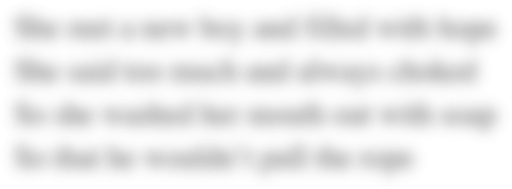# M7A1 Mini Case Ford Company Capital Budgeting Project

The market value of Fords' equity, preferred stock and debt are \$7 billion, \$3 billion, and \$10 billion, respectively. Ford has a beta of 1.8, the market risk premium is 7%, and the risk-free rate of interest is 4%. Ford's preferred stock pays a dividend of \$3.5 each year and trades at a price of \$27 per share. Ford's debt trades with a yield to maturity of 9.5%. What is Ford's weighted average cost of capital if its tax rate is 30%?You are provided with the following information to determine Ford’s weighted average cost of capital that will be used for capital project calculations. As a Finance Analyst for Ford, you will calculate and support the development of its weighted average cost of capital and communicate it to the CFO of Ford. After you have calculated the required data points in the two tables below, provide a brief paper (approximately 300 to 400 words) to the CFO summarizing the weighted average cost of capital you determined for Ford, where they may be risks in the calculation (how the calculation may change over time), how the weighted average cost of capital you calculated should be applied when making cost of capital project decisions, and some scenarios that the CFO should be aware of that may change the weighted average cost of capital at some future point. Your discussion of the scenarios should cover aspects of management, marketing and broader economic factors that may influence the weighted average cost of capital.
Field of study:
Date Due:
Monday, June 25, 2018